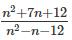Courses

# CAT Previous Year Questions: Quadratic equations CAT Notes | EduRev

## Quant : CAT Previous Year Questions: Quadratic equations CAT Notes | EduRev

The document CAT Previous Year Questions: Quadratic equations CAT Notes | EduRev is a part of the Quant Course Quantitative Aptitude (Quant).
All you need of Quant at this link: Quant

*Answer can only contain numeric values
Try yourself:The number of solutions of the equation |x|(6x2 + 1) = 5x2 is

[TITA 2019]

Try yourself:The product of the distinct roots of |x2 - x - 6| = x + 2 is



Try yourself:What is the largest positive integer such thatis also positive integer?



Try yourself:Let A be a real number. Then the roots of the equation x2 - 4x - log2A = 0 are real and distinct if and only if



Try yourself:The quadratic equation x2 + bx + c = 0 has two roots 4a and 3a, where a is an integer. Which of the following is a possible value of b2 + c?



Try yourself:If u2 + (u−2v−1)2 = −4v(u + v), then what is the value of u + 3v?



Try yourself:The minimum possible value of the sum of the squares of the roots of the equation x2 + (a + 3)x - (a + 5) = 0 is



Offer running on EduRev: Apply code STAYHOME200 to get INR 200 off on our premium plan EduRev Infinity!

## Quantitative Aptitude (Quant)

116 videos|131 docs|131 tests

,

,

,

,

,

,

,

,

,

,

,

,

,

,

,

,

,

,

,

,

,

;